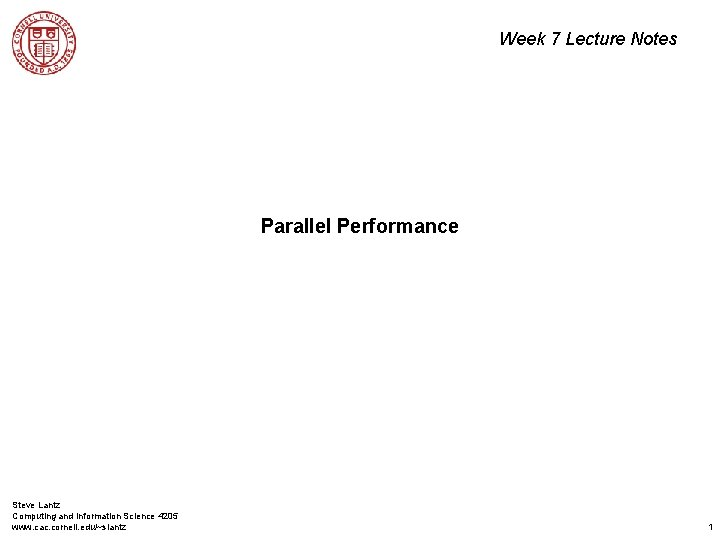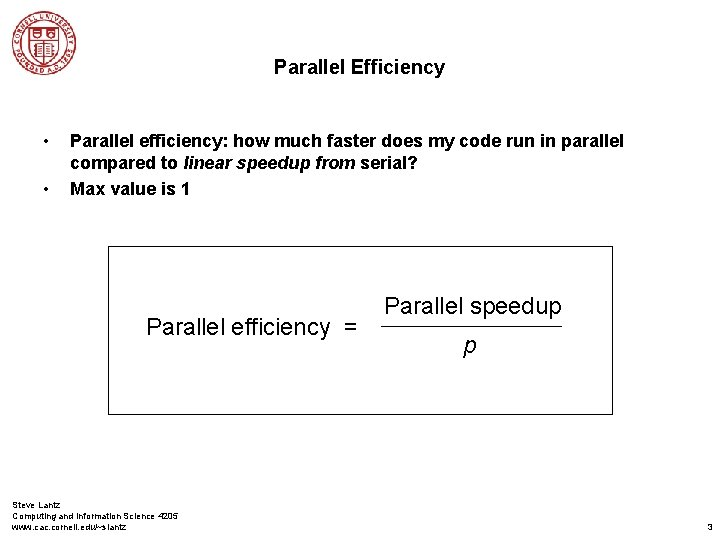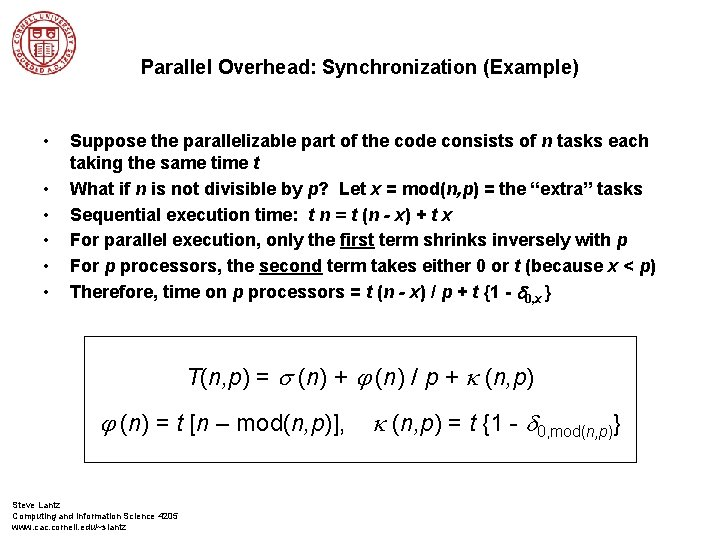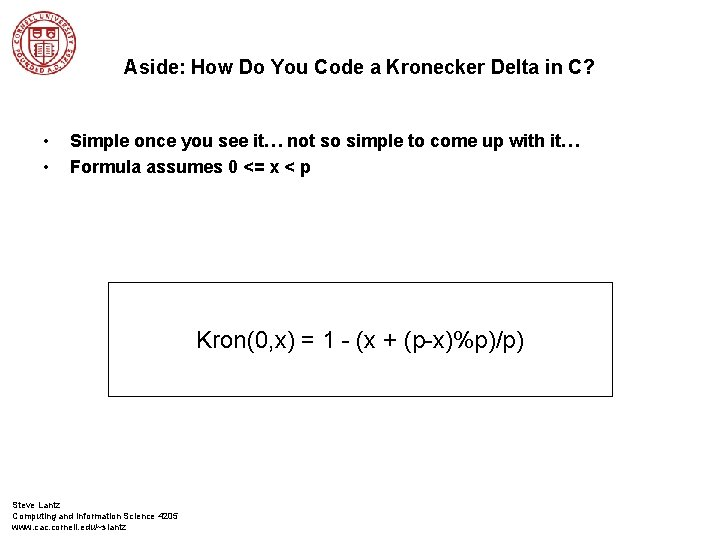# Week 7 Lecture Notes Parallel Performance Steve Lantz

• Slides: 11Week 7 Lecture Notes Parallel Performance Steve Lantz Computing and Information Science 4205 www. cac. cornell. edu/~slantz 1Parallel Speedup • • Parallel speedup: how much faster does my code run in parallel compared to serial? Max value is p, the number of processors Parallel speedup = Steve Lantz Computing and Information Science 4205 www. cac. cornell. edu/~slantz serial execution time parallel execution time 2Parallel Efficiency • • Parallel efficiency: how much faster does my code run in parallel compared to linear speedup from serial? Max value is 1 Parallel efficiency = Steve Lantz Computing and Information Science 4205 www. cac. cornell. edu/~slantz Parallel speedup p 3Model of Parallel Execution Time • • Let n represent the problem size and p be the number of processors Execution time of the inherently sequential portion of the code is s (n) Execution time of the parallelizable portion of the code f (n) / p Overhead is due parallel communication, synchronization is k (n, p) T(n, p) = s (n) + j (n) / p + k (n, p) Steve Lantz Computing and Information Science 4205 www. cac. cornell. edu/~slantz 4Model of Parallel Speedup • • Divide T(n, 1) by T(n, p) Cute notation by Quinn: y (or Greek psi) = parallel speedup y (n, p) = Steve Lantz Computing and Information Science 4205 www. cac. cornell. edu/~slantz s (n) + j (n) / p + k (n, p) 5Amdahl’s Law • • • Parallel overhead k is always positive, so… Can express it in terms of sequential fraction f = s (n) / [s (n) + j (n)] Divide numerator, denominator by [s (n) + j (n)] y (n, p) < Steve Lantz Computing and Information Science 4205 www. cac. cornell. edu/~slantz s (n) + j (n) / p 6Other Expressions for Amdahl’s Law y (n, p) < Steve Lantz Computing and Information Science 4205 www. cac. cornell. edu/~slantz 1 f + (1 - f ) / p p 1 + f (p - 1) 7Parallel Overhead: Synchronization (Example) • • • Suppose the parallelizable part of the code consists of n tasks each taking the same time t What if n is not divisible by p? Let x = mod(n, p) = the “extra” tasks Sequential execution time: t n = t (n - x) + t x For parallel execution, only the first term shrinks inversely with p For p processors, the second term takes either 0 or t (because x < p) Therefore, time on p processors = t (n - x) / p + t {1 - d 0, x } T(n, p) = s (n) + j (n) / p + k (n, p) j (n) = t [n – mod(n, p)], Steve Lantz Computing and Information Science 4205 www. cac. cornell. edu/~slantz k (n, p) = t {1 - d 0, mod(n, p)}Aside: How Do You Code a Kronecker Delta in C? • • Simple once you see it… not so simple to come up with it… Formula assumes 0 <= x < p Kron(0, x) = 1 - (x + (p-x)%p)/p) Steve Lantz Computing and Information Science 4205 www. cac. cornell. edu/~slantzParallel Overhead: Jitter (Example) Steve Lantz Computing and Information Science 4205 www. cac. cornell. edu/~slantzCommunication • • Latency Bandwidth Steve Lantz Computing and Information Science 4205 www. cac. cornell. edu/~slantz